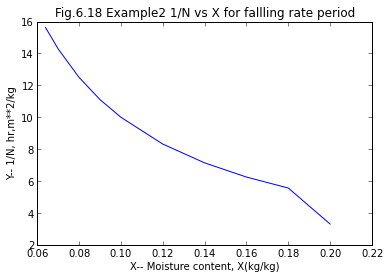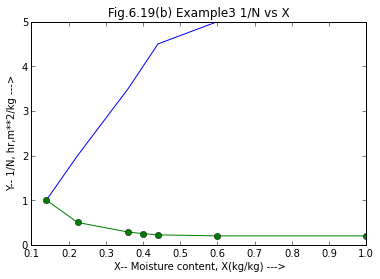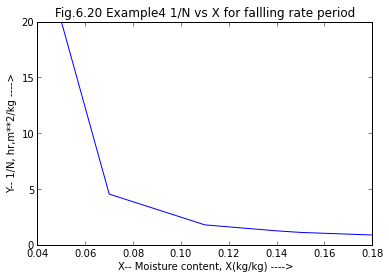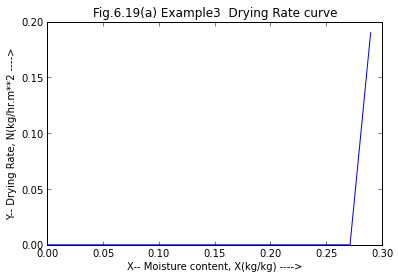Chapter 6 : Drying¶

Example 6.1¶

In :

H1=.005;                        #humidity of incoming air per kg of dry air
T1=25.;                          #wet bulb temperature

print "\n  the solid temp. correspond to wbt and they are 23, 27,32 and 34  degree respectively"

#part(ii)
Ybar=.032;                 #kg water/kg dry air#final moist air condotions
T2=42.;                    #dry bulb temperature
Mair=28.84;               #molecular weight of air
Mwater=18;                  #molecular weight of water
pt=1.013*10**5;            #total pressure in pascal
Vh=8315*((1/Mair)+(Ybar/Mwater))*((T2+273)/pt);
r=300;                    #flow rate of moist air leaving the dryer
a=r*60/Vh;               #amount of dry air leaving /hr
w=a*(Ybar-0.005);         # water removed /hr

# Result
print "\n the water removed /hr is :%fkg /hr"%w

#end
the solid temp. correspond to wbt and they are 23, 27,32 and 34  degree respectively

the water removed /hr is :515.648113kg /hr

Example 6.2¶

In :

%matplotlib inline

Ls=262.5;                   #mass of bone dry solid ais the drying surface
A=262.5/8;                 #both upper surafce and lower surface are exposed
Nc=0.3;                   #in kg/m**2*hr
x2=.06;                  #moisture content on wet basis  finally after drying
x1=.25;                 #moisture content on wet basis  finally after drying
Xcr=0.20;              #crtical moisture content
X1=x1/(1-x1);                     #moisture content on dry basis  intially
X2=x2/(1-x2);                    #moisture content on dry basis  finally after drying
Xbar=0.025;                     #equillibrium moisture

# Calculation
t1=Ls/(A*Nc) *(X1-Xcr);       #so for constant rate period

#for falling rate period we find time graphically
p = [.20 ,.18, .16, .14, .12, .10, .09, .08, .07, .064];
a = [3.3, 5.56, 6.25, 7.14, 8.32, 10.00, 11.11, 12.5, 14.29, 15.625];
from matplotlib.pyplot import *

# Result
plot(p,a);
title("Fig.6.18 Example2 1/N vs X for fallling rate period");
xlabel("X-- Moisture content, X(kg/kg)");
ylabel("Y-- 1/N, hr,m**2/kg");

Area=1.116;                #area under the curve
t2=Area *Ls/A;            #falling rate period we find time graphically
ttotal=t1+t2;            #total time for drying
print "\n the total time for drying the wet slab on wet basis is :%f min"%ttotal
#end
Welcome to pylab, a matplotlib-based Python environment [backend: module://IPython.zmq.pylab.backend_inline].

the total time for drying the wet slab on wet basis is :12.483556 minExample 6.3.a¶

In :

#part(i)

# Variable Declaration
p = [1.0,0.6,.44,0.4,.36,.224, 0.14];
a = [5.0,5.0, 4.5, 4.0,  3.5,  2.00,  1.00];

i=0;                            #looping for calc. of 1/N
t = [0,0,0,0,0,0,0]

# Calculation
while(i<7):                     #looping begins
t[i]=1./(a[i]);
i=i+1;

from matplotlib.pyplot import *

# Result
plot(p,a);
title("Fig.6.19(a) Example3  Drying Rate curve");
xlabel("X-- Moisture content, X(kg/kg) ---->");
ylabel("Y-- Drying Rate, N(kg/hr.m**2 ---->");
#xset('window',1);
plot(p,t,"o-");
title("Fig.6.19(b) Example3 1/N vs X");
xlabel("X-- Moisture content, X(kg/kg) --->");
ylabel("Y-- 1/N, hr,m**2/kg --->");
show()
#from X=0.6 to 0.44 ,falling rate is non linear and from X=.44 to .14 falling rate is linear

print "\n from the graph we get critical moisture content as 0.6 kg moisture/kg dry solid"

#endfrom the graph we get critical moisture content as 0.6 kg moisture/kg dry solid

Example 6.3.b¶

In :

# Variable Declaration
#part(ii)
w1=5.;                           #wet of wet solid
c1=.5/(1-.5);                   #moisture content per kg wet solid
w2=5.*0.5;                       #moisture for 5kg wet solid
w3=w1-w2;                       #weight of dry solid
xbar=0.05;                      #equillibrium moisture content
Xbar=xbar/(1-xbar);             #equillibrium moisture content
Ls=2.5;                        #mass of bone dry solid ais the drying surface
A=5.;                          #both upper surafce and lower surface are exposed
Nc=0.6;                        #in kg/m**2*hr
#from X=0.6 to 0.44 ,falling rate is non linear and from X=.44 to .14 falling rate is linear
X2=.15/(1-.15);
Xcr=.6;                      #kg moisture per kg dry solid
#so we can find time fro drying from 0.6 to .44 graphically and then for X=.44 to .1765
X1=1.;                      #moisture content on dry basis  intially

# Calculation
t1=Ls/(A*Nc) *(X1-Xcr);        #time taken for constant drying rate(fromX=1 to .6)
X1=.44;                      #moisture content on dry basis
import math
t2=(Ls/(A*Nc))*((Xcr-Xbar)*math.log((X1-Xbar)/(X2-Xbar)));
t3=0.0336*Ls/Nc;              #fro graph we get from X=.6 to .44
ttotal=t1+t2+t3;                 #total time for drying the wet slab

# Result
print "\n the total time for drying the wet slab to 15 percent moisture on wet basis is :%f min"%(ttotal*60)

#end
the total time for drying the wet slab to 15 percent moisture on wet basis is :59.610778 min

Example 6.4¶

In :
#let Ls/A=p
# Variable Declaration
p=48.;                   #mass of bone dry solid ais the drying surface
v=1.5*1.5*.5;           #volume of material
Nc=1.22;                #in kg/m**2*hr
Xcr=0.2;                #crtical moisture content
X1=0.25;              #moisture content on dry basis  intially
X2=0.08;               #moisture content on dry basis  finally after drying
Xbar=0.025;           #equillibrium moisture

#tbar=(Ls/(A*Nc))*((Xcr-Xbar)*log((Xcr-Xbar)/(X2-Xbar)));
# Calculation
t1=p/(Nc) * (X1-Xcr);    #time taken for constant drying rate period

# equillibrium relation is given under
p = [.18,.15,.14,.11,.07,.05];
a = [.8772,1.11,1.25,1.7857,4.545,20];
from matplotlib.pyplot import *

# Result
plot(p,a);
title("Fig.6.20 Example4 1/N vs X for fallling rate period");
xlabel("X-- Moisture content, X(kg/kg) ---->");
ylabel("Y-- 1/N, hr,m**2/kg ---->");

a=14*.025*1;         #area under the curve
t2=a*48;            #time taken for varying drying period
ttotal=t1+t2;       #total time taken
print "\n total time for drying the material from 25 to 8 percent moisture under same drying conditions is :%f hr"%(ttotal)

#end
total time for drying the material from 25 to 8 percent moisture under same drying conditions is :18.767213 hrExample 6.5.a¶

In :

# Variable Declaration
w=[5.314,5.238,5.162,5.124,5.048,4.972,4.895,4.819,4.743,4.667,4.524,4.468,4.426,4.340,4.120]
t=[0.0,0.4,0.8,1.0,1.4,1.8,2.2,2.6,3.0,3.4,4.2,4.6,5.0,6.0]
#part(i)
x=4.120;                            #weight of the dried material
print "\n moisture content (dry basis) "
i=0;
p = []                        #looping starts

# Calculation
while(i<15):                        #calculation of moisture content
p.append((w[i]-x)/x);
print "\n     :%f"%p[i]
i=i+1;

print "Drying rate kg/hr*m**2"
i=1;
a = []
for i in range(15):
a.append(0)

while(i<14):
a[i] =((p[i-1]-p[i])*4.12/(t[i]-t[i-1]))
print "  %f "%a[i]
i=i+1;
from matplotlib.pyplot import *
a=.19;
a=0;

# Result
print "\n\n from the above data it is clear that  critical moisture content Xcr=0.11"
plot(p,a);
title("Fig.6.19(a) Example3  Drying Rate curve");
xlabel("X-- Moisture content, X(kg/kg) ---->");
ylabel("Y-- Drying Rate, N(kg/hr.m**2 ---->");
show()
#end
moisture content (dry basis)

:0.289806

:0.271359

:0.252913

:0.243689

:0.225243

:0.206796

:0.188107

:0.169660

:0.151214

:0.132767

:0.098058

:0.084466

:0.074272

:0.053398

:0.000000
Drying rate kg/hr*m**2

from the above data it is clear that  critical moisture content Xcr=0.11Example 6.5.b¶

In :

# Variable Declaration
#part(ii)
w1=4.934;                    #weight after two hours
w0=5.314;                   #initial weight
w2=w0-w1;                  # water evaporated in 2 hrs
H1=.01;                   #humidty of incoming air
H2=.03;                  #humidity of leaving air
yout=.03;
yin=.01;

# Calculation
Gs=w2/(yout- yin);      #water carried away

# Result
print "\n the amount of air required in 2hours is :%f kg"%Gs
#end
the amount of air required in 2hours is :19.000000 kg

Example 6.5.c¶

In :

# Variable Declaration
#part(iii)
#let us choose the consistency of 11 and 13 readings
Xbar=0;                     #equillibrium moisture content
Ls=4.12;                    #mass of bone dry solid ais the drying surface
A=1.;                       #both upper surafce and lower surface are exposed
Nc=0.19;                   #in kg/m**2*hr
X1=.098;                   #moisture content on dry basis  intially
Xcr=.11;                   #kg moisture per kg dry solid
X2=0.074;                   #moisture content on dry basis  finally
# Calculation
import math
tfall=(Ls/(A*Nc))*((Xcr-Xbar)*math.log((X1-Xbar)/(X2-Xbar)));

# Result
print "\n from this data we get time as :%f hour"%tfall
print "\n the actual time is 0.8 hours"
#end
from this data we get time as :0.670026 hour

the actual time is 0.8 hours

Example 6.6¶

In :

# Variable Declaration
Xcr=0.55;             #crtical moisture content
X1=1;               #moisture content on dry basis intially
X2=.1;              #moisture content on dry basis finally after drying
Xbar=.06;            #equillibrium moisture
#since eqn 1 is tobe divided by eqn 2 so let the value of Ls/A*Nc be = 1 as it will be cancelled
p=1;                  #let Ls/A*Nc be =p
#p=poly(,'p');        #calc. of time 1
tbar=1;                  #since the eqns are independent of tbar

# Calculation
import math
t1=0.5960514   #roots(tbar- p*((X1-Xcr)+(Xcr-Xbar)*math.log((Xcr-Xbar)/(X2-Xbar)))); #------eqn1
X2bar=.16;
#p=poly(,'p');        #calc. of time 2
t2=0.8138516   #roots(tbar- p*((X1-Xcr)+(Xcr-Xbar)*math.log((Xcr-Xbar)/(X2bar-Xbar))));#------eqn2

#let t1/t2 be = k
k=t1/t2;
ans=1./k-1;            #reduction in time for drying

# Result
print "\n the reduction in time for drying is :%f percent"%(ans*100)
#end
the reduction in time for drying is :36.540506 percent

Example 6.7¶

In :

#Ls/ A*Nc is unknown;
# Variable Declaration
Xcr=0.14;             #crtical moisture content
X1=.3/(1-.3);         #moisture content on dry basis intially
X2=0.1/(1-0.1);       #moisture content on dry basis finally after drying
Xbar=.04;            #equillibrium moisture
tbar=5;              #time needed to dry from 30 to 6 percent on bone dry basis

#let Ls / A*Nc be = p
#p=poly(,'p');        #calc. of Ls / A*Nc be = p value
# Calculation  and  Result
x= 15.495992    #roots(tbar-p * ((X1-Xcr)+(Xcr-Xbar)*log((Xcr-Xbar)/(X2-Xbar))));
print "\n the value of Ls/ A*Nc is :%f"%x
import math
#new X1 AND X2 are now given as follows
X1=0.3/(1-.3);              #new moisture content on dry basis intially
X2=0.064;                 #new moisture content on dry basis finally after drying
tbar=x * ((X1-Xcr)+(Xcr-Xbar)*math.log((Xcr-Xbar)/(X2-Xbar)));
print "\n the time for drying the sheets from 30 to 6  percent moisture under same drying conditions is :%f hr"%tbar

#end
the value of Ls/ A*Nc is :15.495992

the time for drying the sheets from 30 to 6  percent moisture under same drying conditions is :6.683159 hr

Example 6.8.a¶

In :

# Variable Declaration
Ls=1000.;              #mass of bone dry solid ais the drying surface
A=55.;                 #both upper surafce and lower surface are exposed
v=.75;                #velocity of air
Nc=.3*10**-3;          #in kg/m**2*s
x2=.2;                #moisture content on wet basis  finally after drying
Xcr=0.125;             #crtical moisture content
X1=0.15;              #moisture content on dry basis  intially
X2=0.025;             #moisture content on dry basis  finally after drying
Xbar=0.0;        #equillibrium moisture
import math

# Calculation
tbar=(Ls/(A*Nc))*((X1-Xcr)+(Xcr-Xbar)*math.log((Xcr-Xbar)/(X2-Xbar)));

# Result
print "the time for drying the sheets from .15 to .025 kg water /kg of dyr solid moisture under same drying conditions is :%f hour"%(tbar/3600);

#end
the time for drying the sheets from .15 to .025 kg water /kg of dyr solid moisture under same drying conditions is :3.807740 hour

Example 6.8.b¶

In :

# Variable Declaration
Ls=1000.;              #mass of bone dry solid ais the drying surface
A=55.;                 #both upper surafce and lower surface are exposed
v=.75;                #velocity of air
Nc=.3*10**-3;          #in kg/m**2*s
x2=.2;                #moisture content on wet basis  finally after drying
Xcr=0.125;             #crtical moisture content
X1=0.15;              #moisture content on dry basis  intially
X2=0.025;             #moisture content on dry basis  finally after drying
Xbar=0.0;        #equillibrium moisture
tbar=3.8077;       #time to dry material ,calculated from previous part
V1=.75;                #old velocity
V2=4.;                  #new velocity
import math

# Calculation
Nc2=Nc*(V2/V1)**.71;    #in kg/m**2*s
t2=(Ls/(A*Nc2))*((X1-Xcr)+(Xcr-Xbar)*math.log((Xcr-Xbar)/(X2-Xbar)));    #if air velocity is increased to 4
t=tbar-t2/3600;            #time saved

# Result
print "\n the time saved , if air velocity is increased to 4 m/s: %f"%t

#end
the time saved , if air velocity is increased to 4 m/s: 2.647593

Example 6.9¶

In :

x1=.75;                #moisture content on wet basis
xbar=0.1;                #equilllibrium moisture on dry basis
xcr=0.6;                #critical moisture content
Ls=0.90;                #mass of bone dry solid ais the drying surface
A=0.3*0.3*2;            #both upper surafce and lower surface are exposed
#A*Nc=10**-4;
x2=.2;                #moisture content on wet basis  finally after drying

# Calculation
Xcr=0.6/0.4;          #crtical moisture content
X1=3;                 #moisture content on dry basis  intially
X2=0.25;              #moisture content on dry basis  finally after drying
Xbar=0.1/0.9;        #equillibrium moisture
import math
tbar=Ls/(10**-4) * ((X1-Xcr)+(Xcr-Xbar)*math.log((Xcr-Xbar)/(X2-Xbar)));

# Result
print "\n the time for drying the sheets from 75 to 25 percent moisture under same drying conditions is :%f hr"%(tbar/3600);

#end
the time for drying the sheets from 75 to 25 percent moisture under same drying conditions is :11.745087 hr

Example 6.10¶

In :

# Variable Declaration
Xcr=0.16;             #crtical moisture content
X1=.33;               #moisture content on dry basis intially
X2=0.09;              #moisture content on dry basis finally after drying
Xbar=.05;            #equillibrium moisture
tbar=7;              #time needed to dry from 33 to 9 percent on bone dry basis
import math
#let Ls / A*Nc be = p
#p=poly(,'p');        #calc. of Ls / A*Nc be = p value
# Calculation
x= 24.886579  #roots(tbar-p * ((X1-Xcr)+(Xcr-Xbar)*math.log((Xcr-Xbar)/(X2-Xbar))));

#new X1 AND X2 are now given as follows
X1=0.37;              #new moisture content on dry basis intially
X2=0.07;               #new moisture content on dry basis finally after drying
tbar=x * ((X1-Xcr)+(Xcr-Xbar)*math.log((Xcr-Xbar)/(X2-Xbar)));

# Result
print "\n the time for drying the sheets from 33 to 9  percent moisture under same drying conditions is :%f hr"%tbar

#end
the time for drying the sheets from 33 to 9  percent moisture under same drying conditions is :9.892970 hr

Example 6.11¶

In :

# Variable Declaration
d=.22;                    #density of dry pulp in g/cc;
x1=.65;                #moisture content on wet basis
x2=.3;                #moisture content on wet basis
Ls=2.5;                #mass of bone dry solid ais the drying surface in kg
A=1.5*1.5*2;            #both upper surafce and lower surface are exposed
v=1.5*1.5*.5;           #volume of material
Nc=1.4;                 #in kg/m**2*hr
Xcr=1.67;              #crtical moisture content
# Calculation
X1=x1/(1-x1);                 #moisture content on dry basis  intially
X2=x2/(1-x2);              #moisture content on dry basis  finally after drying
Xbar=0.0;             #equillibrium moisture
import math

tbar=(Ls/(A*Nc))*((X1-Xcr)+(Xcr-Xbar)*math.log((Xcr-Xbar)/(X2-Xbar)));

# Result
print "\n the time for drying the sheets from 65 to 30 percent moisture under same drying conditions is :%f hour"%tbar

#end
the time for drying the sheets from 65 to 30 percent moisture under same drying conditions is :0.975613 hour

Example 6.12¶

In :
# Variable Declaration
d=.22;                    #density of dry pulp in g/cc;

Ls=1.125*10**-2*.22*10**3;                #mass of bone dry solid ais the drying surface
A=1.5*1.5*2;            #both upper surafce and lower surface are exposed
v=1.5*1.5*.5;           #volume of material
Nc=1.4;                #in kg/m**2*hr
x2=.2;                #moisture content on wet basis  finally after drying
Xcr=0.46;              #crtical moisture content
X1=0.15;                 #moisture content on dry basis  intially
X2=0.085;              #moisture content on dry basis  finally after drying
Xbar=0.025;        #equillibrium moisture
import math
#tbar=(Ls/(A*Nc))*((Xcr-Xbar)*log((Xcr-Xbar)/(X2-Xbar)));

# Calculation
tbar=(Ls/(A*Nc))*((Xcr-Xbar)*math.log((X1-Xbar)/(X2-Xbar)));

# Result
print "\n the time for drying the sheets from 15 to 8.5 percent moisture under same drying conditions is :%f min"%(tbar*60)

#end
the time for drying the sheets from 15 to 8.5 percent moisture under same drying conditions is :7.525805 min

Example 6.13¶

In :

# Variable Declaration
import math
Xcr=0.14;             #crtical moisture content
x1=0.3;                #moisture content on wet basis
x2=0.1;                #moisture content on wet basis
X1=x1/(1-x1);               #moisture content on dry basis intially
X2=x2/(1-x2);              #moisture content on dry basis finally after drying
Xbar=0.04;            #equillibrium moisture
tbar=5;              #time needed to dry from 30 to 10 percent on bone dry basis

#let Ls / A*Nc be = p
#p=poly(,'p');        #calc. of Ls / A*Nc be = p value
x= 15.495992 #roots(tbar-p * ((X1-Xcr)+(Xcr-Xbar)*math.log((Xcr-Xbar)/(X2-Xbar))));

#new X1 AND X2 are now given as follows
# Calculation
x1=.3;                #new moisture content on wet basis
x2=0.06;                #new moisture content on wet basis
X1=x1/(1-x1);              #new moisture content on dry basis intially
X2=x2/(1-x2);              #new moisture content on dry basis finally after drying
tbar=x * ((X1-Xcr)+(Xcr-Xbar)*math.log((Xcr-Xbar)/(X2-Xbar)));

# Result
print "\n the time for drying the sheets from 30 to 10  percent moisture under same drying conditions is :%f hr"%tbar

#end
the time for drying the sheets from 30 to 10  percent moisture under same drying conditions is :6.694188 hr

Example 6.14¶

In :

d=450.;                    #density of dry pulp in kg/m**3;
thickness=0.05;           #thickness in m**2
Ls=d*thickness;           #mass of bone dry solid ais the drying surface
A=1.;                    #area in m**2
v=1*5*10**-2;           #volume of material
Nc=4.8;                #in kg/m**2*hr
xcr=.2;
xbar=0.02;
x1=.45;                #new moisture content on wet basis
x2=0.05;                #new moisture content on wet basis

# Calculation
X1=x1/(1-x1);              #new moisture content on dry basis intially
X2=x2/(1-x2);              #new moisture content on dry basis finally after drying
Xbar=xbar/(1-xbar);              #crtical moisture content
Xcr=xcr/(1-xcr);        #equillibrium moisture
import math
#tbar=(Ls/(A*Nc))*((Xcr-Xbar)*log((Xcr-Xbar)/(X2-Xbar)));

tbar=Ls/(A*Nc) * ((X1-Xcr)+(Xcr-Xbar)*math.log((Xcr-Xbar)/(X2-Xbar)));

# Result
print "\n the time for drying the sheets from 45 to 5 percent moisture under same drying conditions is :%f min"%tbar

#end
the time for drying the sheets from 45 to 5 percent moisture under same drying conditions is :4.776612 min

Example 6.15¶

In :

# Variable Declaration
t1=20.;                   #ambient air temperature
t2=70.;                   #exhaust air temperature
r1=150.;                  #evaporation of water
r2=.25;                 #outlet solid moisture content
t3=15.;                #inlet solid temperature
t4=65.;                  #outlet solid temperature
p=5.;                   #power demand in KW
h=18.;                  #heat loss in kj

h1=1.;                    #mean specific heat of dry air in kj/kg*K
h2=1.25;                    #mean specific heat of dry material in kj/kg*K
h3=4.18;                    #mean specific heat of moisture in kj/kg*K
e=2626.;                    #enthalpy of saturated water vapour in kj/kg

# Calculation
#basis is 1hr
a1=r1*h3*(t4-t3);          #heat required for heating 150 kg of water from 15 to 65
a2=r1*e;                   #heat required for 150 kg water evaporation
a3=2000*h1*(t2-t1);        #heat required for heating air from 20 to 70
a4=r2*h3*(t4-t3);          #heat required for heating moisture from 15 to 65
a5=120*h2*(t4-t3);         #heat required for heating dry solid from 15 to 65
hlost=h*3600;             #heat lost in kj
total=(a2+a3+a4+a5+hlost)/3600.;    #total heat lost

# Result
print "\n :%f  kW of heat required for 2000kg/hr of dry air"%total
ans1=a2+a1;            #heat needed for evaporation
print "\n heat needed fro evaporation is :%f"%(ans1/3600)
ans2=(ans1/3600.)/total;    #fraction of this heat needed for evaporation
print "\n fraction of this heat needed for evaporation:%f"%(ans2)

#end
:157.292292  kW of heat required for 2000kg/hr of dry air

heat needed fro evaporation is :118.125000

fraction of this heat needed for evaporation:0.750990

Example 6.16¶

In :

m1=.12;                    #initial moisture content
dT=85.;                    #product of 85 degree is used in design purpose
U=1700.;                   #overall heat transfer coefficient
m2=.4;                    #final moisture content
r=20.;                   #production rate
#4 kg of moisture is present in 100 kg product
# Calculation
t=4*20./100;            # moisture content in 20 kg moisture
w=20-t;                #dry solid weight
i=w*m1/(1-m1);        #initial moisture content
j=i-t                #water evaporated
ds=2296.1;           #latent heat for vaporisation at 85 degree in kj/kg
h=j*ds;                #heat required (assuming th esolid mix. enters at 85)
#U*A*dT = j*ds
A=h/(U*dT);            #surface area of the roller required to produce aproduction rate of 20 kg product per hour

# Result
print "\n surface area of the roller required to produce aproduction rate of 20 kg product per hour:%f m**2"%(A/3.600);

#end
surface area of the roller required to produce aproduction rate of 20 kg product per hour:0.008025 m**2

Example 6.17¶

In :

# Variable Declaration
r=7.5*10**-5;                    #constant drying rate in kg/s
A1=.3*.3**2;                    # area of the sppecimen
Nc=r/A1;                        #drying rate
# Calculation
Xcr=.15/0.85;                    #.15 is the critical moisture content
Xo=.25/.75;                    #.25 is the initial moisture content
Xfinal=.02/0.98;               #.02 is the final moisture content
Xbar=0;                      #equillibrium moisture content
A=1.2*.6*2;                 #area of the new solid
Ls=28.8;                     #bone dry weight of new solid
v1=.3*.3*.006;               #volume of the old solid;
v2=.6*1.2*.012;              #volume of the new solid
w2=1.8;                     #weight of the old solid
w3=864*10**-5*1.8*10**-5/54;   #weight of the bone dry solid

#Nc is prporional to =(t-ts) = (G)**0.71---- whrere G is the mass flow rate
v1=3.;                      #old velocity
Tg=52.;                     #old dry bulb temperature
Tw=21.;                     #wet bulb temperature
H=.002;                    #humidity
SH=0.015;                  #saturated humidity
vnew=5.                    #new velocity
Tgnew=66.;                 #new DBT
Twnew=24.;                 #new WBT
Hnew=.004;               #new humidity
SH=.020;                #new satuurated humidity
import math
#hence drying rate of air under new condition
Nc=4.167*10**-4*((vnew/v1)*(273+Tg)/(273+Tgnew))**0.71 * ((.019-H)/(.015-H));#drying rate of air under new condition in kg/m**2*s
DT=Ls/(A*Nc) * ((Xo-Xcr)+(Xcr-Xbar)*math.log((Xcr-Xbar)/(Xfinal-Xbar)));

# Result
print "\n the time for drying the sheets from 25 to 2 percent moisture under same drying conditions is :%f hours"%(DT/3600)

#end
the time for drying the sheets from 25 to 2 percent moisture under same drying conditions is :3.929236 hours